Science of Lattices and CrystalsBefore I get started: These two modules give a first idea about lattices and crystals without all the formal stuff coming up here:

Bravais LatticesAny crystal lattice can be described by giving a set of three base vectors a1, a2, a3. A lattice is formed by generating an infinity of translations vectors T = ua1 + va2 + wa3 with u, v, w, = integers. The end points of all possible translations vectors define the lattice as a periodic sequence of points in space.If you have some lattice and move it by any translation vector you care to construct, you have exactly the same lattice once more. In other words: crystal lattices show a translation symmetry! For a long time, the words "crystal" and translation symmetry were seen as obvious synonyms - until the discovery of quasi-crystals in 1982!Unfortunately, one and the same lattice can be defined by many different sets of vector triples as illustrated right below.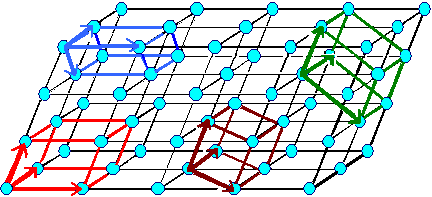Definging a lattice by a vector triple a1, a2, a3. There are, however, many different vector triples that define the same lattice.It is far easier to use some special lattices instead of just one general type. The thing to do is to go for symmetries as the distinguishing criterion. That's what Bravais did, showing that with 14 Bravais lattices all possible cases can be represented. All material scientists know that "magic" number 14 but very few know how it is derived. I don't know details either but I know it is an exercise in set theory.
Note that a lattice is a mathematical construct, a succession of (infinitely small) mathematical points in space. A perfect drawing of such a lattice thus would show nothing at all. Instead of points, I use little blue spheres here. They are connected with lines but only to "guide the eye".
These blue spheres are not representing atoms when a lattice is shown. More about figures to lattices and crystals in this link.If one wants to make a crystal, one assigns a so-called base of atoms to a lattice point. If that base happens to consist of just one atom or element, we make an element crystal. A schematic figure of such a crystal with one atom per lattice point then looks exactly like the schematic representation of a lattice, causing no end of confusion. It is nevertheless something completely different; see below.
Note that mother nature has not made a cubic primitive element crystal.

Bravais Lattices and Their Parameters

 Cubic a1= a2 = a3 = a = lattice constant     a = b = g = 900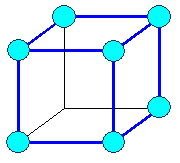cubic primitive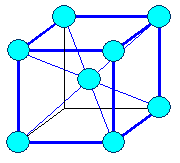cubic body centered (bcc)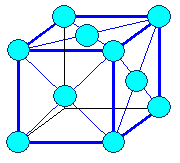cubic face centered (fcc) Tetragonal a1= a2 ¹ a3     a = b = g = 900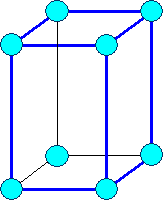tetragonal primitive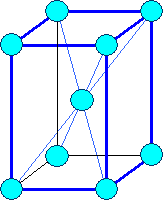tetragonal body centered Hexagonal a1= a2 ¹ a3     a = b = 900, g = 1200     Typical: a3 = c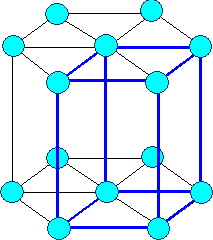Hexagonal (hex) (expanded to show hex symmetry) Rhombohedral or Trigonal a1= a2 = a3     a = b = g ¹ 900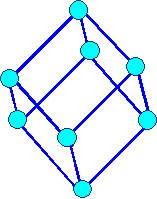rhombohedral Orthorhombic a1 ¹ a 2 ¹ a3     a = b = g = 900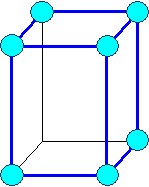orthorhombic primitiv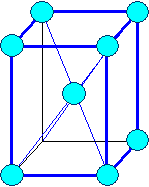orthorhombic body centered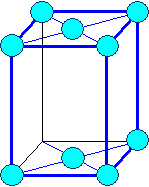orthorhombic base face centered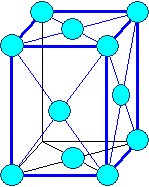orthorhombic face centered Monoclinic a1 ¹ a2 ¹ a3     a = b = 900,     g ¹ 900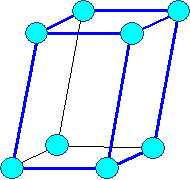monoclinic primitive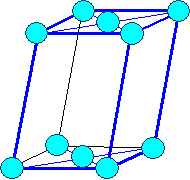monoclinic base face centered Triclinic a1 ¹ a2 ¹ a3     a ¹ b ¹ g ¹ 900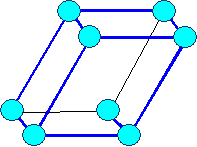triclinic Bravais lattices (on occasion only "visible" lattice points (= blue circles) are shown)

Describing Directions and Planes by Miller IndicesWorking with lattices and crystals produces rather quickly the need to describe certain directions and planes in a simple and unambigous way. Stating that an elemental face-centered cubic crystal can be made by assigning one atom to any lattice point found on "that plane that runs somehow diagonally through the unit cell" just won't do it.
So William Hallowes Miller invented a system with a lot of power for doing that in 1839. What we do is to describe any direction or any plane by three integer numbers, called Miller indices.How to derive the Miller indices of a certain direction or plane is easy. Here is the recipe for directions (in 2 dimensions for simplicity); the figure below illustrates it:
• Start the desired direction from the origin.
• Express the direction as a vector given in integer multiples u, v, w of the base vectors.
• Make sure the three integers have the smallest possible value.
• Write the direction as [u v w] or <u v w> (we won't concern us here with the subtleties involved in using two kinds of brackets).
• Negative integer values are written with a dash on top of the number instead of the conventional "-" sign. (not possible in simple HTML)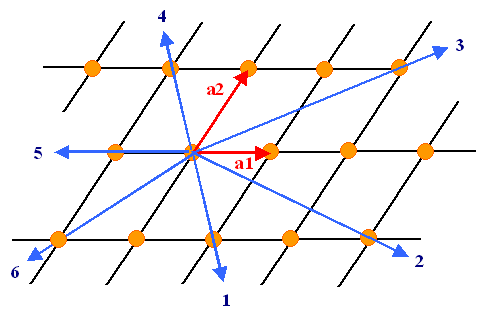Six directions in a (2-dim) lattice and their Miller indicesGetting Miller indices for planes is a bit more involved. Here is how it's done; the figure below gives examples:
• Put the origin not on the plane but on a neighboring plane.
• Find the intersection points h', k', and l' of the plane with the (extended) base vectors. If there is none, the value is ¥.
• Form the reciprocal values of h', k', and l' and call them h, k, and l. If, for example, h' = ¥, you have h = (1/h') = 0.
• The Miller indices of the plane to be indexed then are {hkl} or (hkl).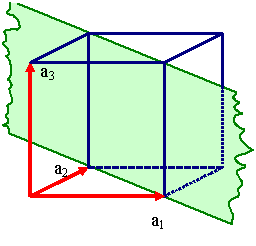Cubic lattice Intersections at 1, 1, ¥ Indices (110)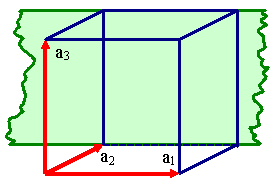Cubic lattice Intersections at ¥, 1, ¥ Indices (010)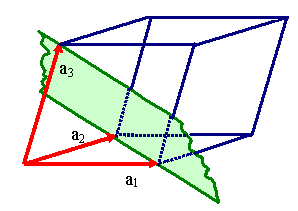Triclinic lattice Intersections at 1, 1, 1 Indizes (111) Miller Indices for PlanesIf you wonder why this slightly awkward procedure was adopted, the answer is easy: You can use the Miller indices directly in a lot of equations needed for calculating properties of crystals.

From Lattice to CrystalAny crystal can be made following this easy recipe:
1. Pick a Bravais lattice
2. Pick a base, a collection of atoms in a fixed spatial relation (similar and often but not always identical to a molecule of the substance.
3. Put the base in the same way on any lattice point.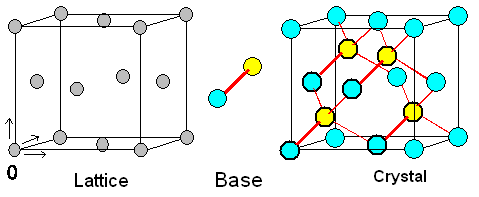How to make a diamond-type crystal The yellow atom of the base is only shown if it is found inside the unit cellThe example above shows how to make a crystal of the diamond type. The base consists of two atoms. In the coordinate system of the lattice unit cell (indicated by arrows), the two atoms have the coordinates (0,0,0) and (¼,¼,¼).
If the two atoms are of the same kind, e.g. silicon, (Si), germanium (Ge), or carbon (C), you get a silicon, germanium or diamond crystal.
If the atoms are different, e.g. from group III or group V of the periodic table, you get most of the compound semiconductors like gallium arsenide (GaAs), or indium phosphide (InP).This looks simple. It is not. It's the point where things get difficult and confusing. Ask yourself for any still simple crystal: how many atoms are there to a lattice plane? How many atoms are in a base?
Below are three crystals, all have an fcc lattice. Different colors of the circles my or may not denote different atoms. Can you figure out the bases? If you can, you're ahead of my average third-term student.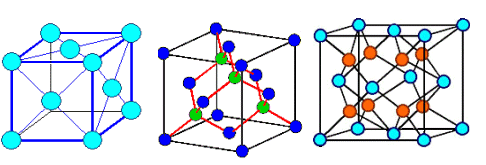Gold, Aluminum, .. Silicon, carbon (diamond), Gallium arsenide (GaAs), InP, .. Zirconium oxide (ZrO2), .. Three different kinds of crystals with the same latticeOne last thought: Crystals in a general sense, meaning an arbitrary base arranged in a periodic way, can be found everywhere; here is an example: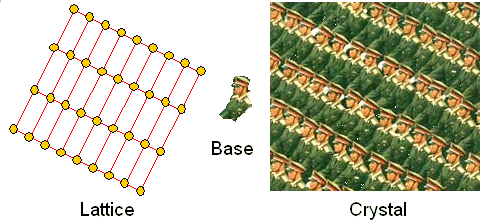Example of general crystal.Periodic Table of the Elements4.2.2 Being IronHistory of Carbon11.2.2 Metallurgy of Celtic SwordsGroup 1 / IA; Alkali GroupGroup 2 / IIA; Alkaline Earth Metals GroupGroup 12 / IIB; Scandium GroupGroup 12 / IIB; Titanium GroupGroup 5 / VB; Vandium GroupGroup VIB; Chromium GroupGroup 7 / VIIB; Manganese GroupGroup 8 - 10 / VIIIB; Iron - Platinum GroupGroup 11 / IB; Copper GroupGroup 12 / IIB; Zinc GroupGroup 13 / IIIA;Group 14 / IVA; Carbon GroupGroup 15 / VA; Nitrogen GroupGroup 16 / VIA; Chalkogenides or Oxygen GroupGroup 18 / VII; Noble GasesGroup 1/ I; HydrogenGroup 3 / IIIB; Lanthanides or "Rare Earths"Group 17 / VIIA; HalogensMagnetismAlloying Elements in Detail8.4.1 MartensiteInhomogeneous DeformationDislocation Science - 1. The BasicsLattice and CrystalCrystal ModelsX-Ray DiffractionPhenomenological Modelling of DiffusionGemstonesBeer and Conquering The WorldQuasi CrystalsScience of DeformationDislocation Science - 2. The RealityPictures of Grain BoundariesDeformation TypesPhase Boundary - Advanced

© H. Föll (Iron, Steel and Swords script)